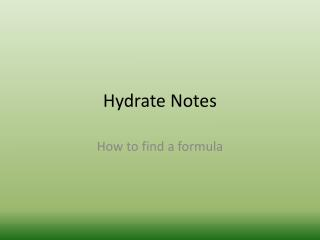DownloadDownload PresentationHydrate Notes

# Hydrate Notes

Télécharger la présentation## Hydrate Notes

- - - - - - - - - - - - - - - - - - - - - - - - - - - E N D - - - - - - - - - - - - - - - - - - - - - - - - - - -
##### Presentation Transcript

1. Hydrate Notes How to find a formula

2. 6 Steps to Find Hydrate Formula(goal is to solve for X) • Write out generic formula: salt · X H₂O • Find mass of water that was heated off: • Wet salt – dry salt or the difference in mass after heating • Convert g of water into moles of water using molar mass of water (round moles to nearest whole #) • Convert g of dry salt into mole of dry salt using the molar mass of the dry salt (round moles to nearest whole #)

3. 6 Steps to Find Hydrate Formula(goal is to solve for X) • If ratio of moles is 1 mole salt to # water skip to step 6…if not go to step 5 5. Divide mole numbers by the smallest mole number 6. X is moles of water…finish formula from step 1.

4. When 380 grams of hydrated copper II sulfate was heated in a crucible, 163.2 g of the anhydrous (dry) compound is left. What is the correct formula of the hydrate? Step 1 CuSO₄· X H₂O Step 2 380 g wet salt – 163.2 g dry salt= 220 g water Step 3 220 g H₂O x (1mol H₂O /18.02g H₂O)= 12 mol H₂O Step 4 163.2 g CuSO₄ x (1 molCuSO₄/ 159.62 g CuSO₄) = 1 molCuSO₄ Step 6 CuSO₄· 12 H₂O

5. 802.5 grams of hydrated zinc sulfate was heated. The change in mass was 352.0 grams. What is the correct formula of the hydrate? Step 1 ZnSO₄· X H₂O Step 2 Change in mass was the mass of water… 802.5 grams wet salt- 352.0 grams water= 450.5 g dry salt Step 3 352.0 g H₂O x (1mol H₂O /18.02g H₂O)= 20 mol H₂O Step 4 450.5 g ZnSO₄ x (1 molZnSO₄/ 161.45 g ZnSO₄) = 3 molZnSO₄ Step 5 20 mol H₂O /3 = 7 H₂O 3 molZnSO₄ / 3= 1 ZnSO₄ Step 6 ZnSO₄· 7 H₂O